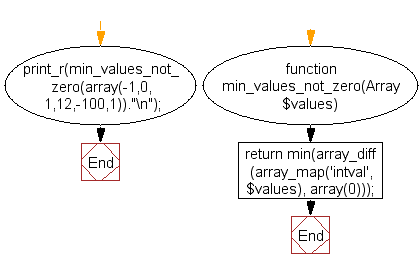﻿ PHP Array Exercise: Return the lowest integer (not 0) from a list of numbers - w3resource# PHP Array Exercises : Return the lowest integer (not 0) from a list of numbers

## PHP Array: Exercise-17 with Solution

Write a PHP function that returns the lowest integer that is not 0.

Sample Solution:

PHP Code:

``````<?php
function min_values_not_zero(Array \$values)
{
return min(array_diff(array_map('intval', \$values), array(0)));
}
print_r(min_values_not_zero(array(-1,0,1,12,-100,1))."\n");
?>
```
```

Sample Output:

```-100
```

Flowchart:PHP Code Editor:

What is the difficulty level of this exercise?

﻿

## PHP: Tips of the Day

PHP: Anonymous recursive PHP functions

In order for it to work, you need to pass \$factorial as a reference

```\$factorial = function( \$n ) use ( &\$factorial ) {
if( \$n == 1 ) return 1;
return \$factorial( \$n - 1 ) * \$n;
};
print \$factorial( 5 );
```

Ref : https://bit.ly/38dj7jm